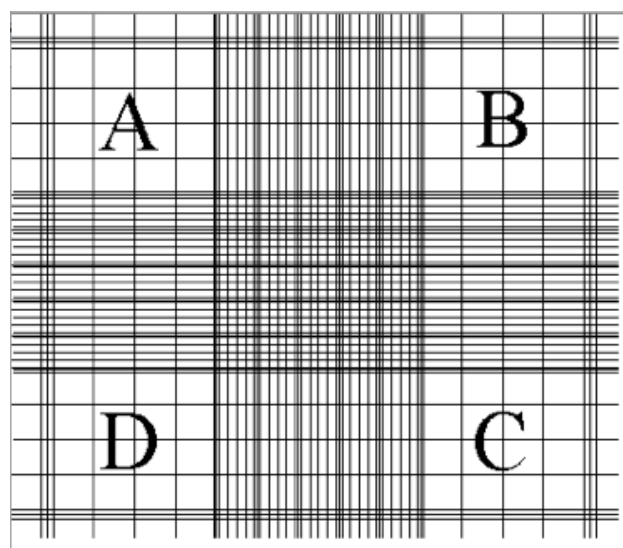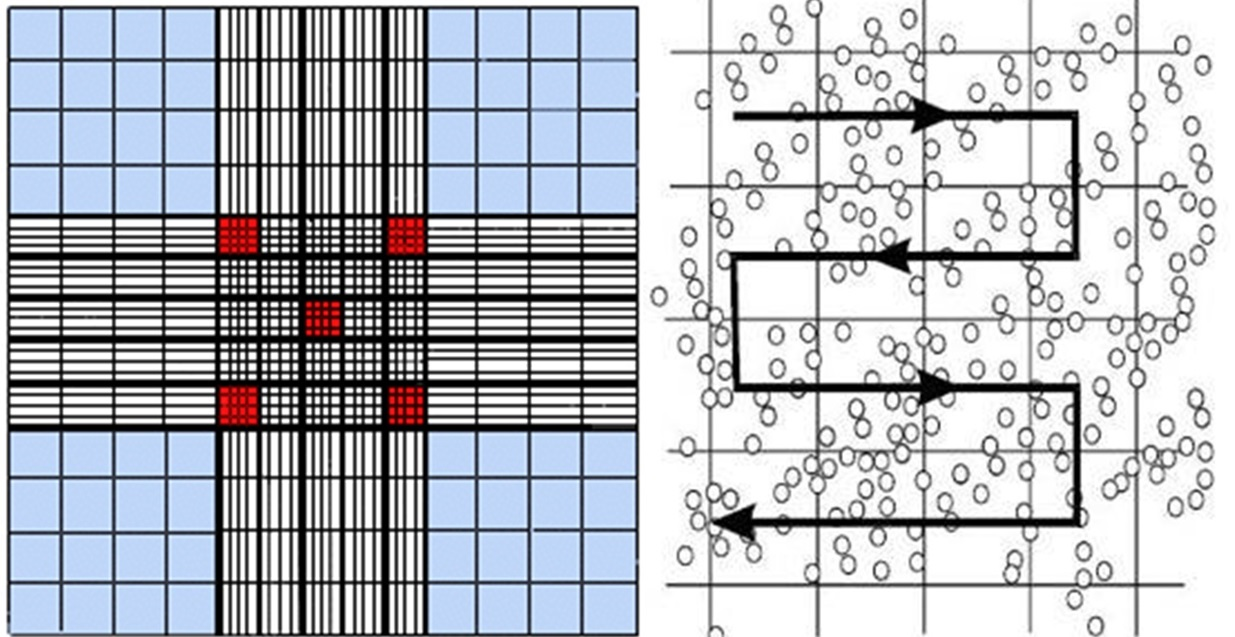# Algae Counting

The algal density determines which counting cell to use. For high density - use the Hemocytometer. If you count less than 5 cells per 1 mm2 (size of big square - i.e. block A, Figure 1) it is low density. For low density - use the Sedgwick cell

Procedure for Hemocytometer:
1) Wipe the middle part and tunnels, and the cover glass
2) Place the cover glass on the counting cell
3) Stir the sample well with a pipet
4) Fill the pipet with the stirred sample
5) Place the tip on one triangle, and ﬁll that chamber with sample
6) Stir the sample well with a pipet
7) Fill the pipet with the stirred sample
8) Place the tip on second triangle, and ﬁll that chamber with sample
9) Wait for a few minutes to let the algae cells time to settle
10) Position the Hemocytometer to start with the lower chamber, ﬁnd the gridded area (Figure 1) set the microscope to X10
11) Set the counter to Zero (0000)
12) Each chamber had 9 big squares, at total count the algae in each of the 18 squares.Each big square is a 1 mm2
A, B, C, & D - each has 16 middle size squares
Center - has 25 middle size squares
Between A-B, A-D, D-C, & C-B - each has 20 middle size squaresFigure 1: The gridded area of the hemocytometer
13) Start at block A at the upper left square - its borders on top and left are 3 lines
14) While scanning all the middle size squares count all the algae in that big square,
move as in Figure 2Figure 2: Direction for counting algae in 1 big square

BORDERS - count only algae that are within the big square’s border. Each big square is separated from its neighbors by 3 lines. The border is the middle line of those three.
15) Record the number of algae cells on the electronic data sheet
16) Proceed to the square between A-D, than to square D, than to the square between D-C, than to the center, than to the square between A-B, than to square B, than to
the square between B-C, than to square C (Figure 1)
17) Count the algae in each of these big squares, record the number you have reached at the end of each square.
18) When you ﬁnished with the ﬁrst chamber, move to the other one.
19) Record in the lab book the total number of algae cells, the number of big squares you counted and the total volume, and the density of algae cells. In total count at least 100 algae cells!!
20) Rinse the hemocytometer and the cover glass with RO water. Wipe to dry with kimwipe
21) Repeat all steps for a second replica
22) If the difference of algae cells density between the duplicates is to high repeat all
steps for the third replica. Don’t count more than 4 replicates.

Procedure for Sedgwick cell: the counting cell is the gridded area
1) Wipe the cell, and the cover glass
2) Stir the sample well with a pipet
3) Fill the pipet with the stirred sample
4) Place the pipet at one corner of the counting cell and start ﬁlling it with the sample moving the pipet toward the other side of the counting cell (start at left bottom corner, move to upper corner and then to the right).
5) Place the cover glass on the counting cell, make sure that the sample ﬁll the entire counting cell without forming air bubbles.
6) Absorb the excess of sample from both sides of the counting cell with a kimwipe.
7) Wait for 5-10 minutes to let the algae cells time to settle
8) Position the Sedgwick cell to start at one of the sides, ﬁnd the grid and set the microscope to X10
9) Set the counter to Zero (0000)
10) The amount of squares need to be counted is determined by the density of algae cells. In total count more than 200 algae cells!! i-very low density (aprox. 5 algae cells in a square): count all squares from left to right, at 3 areas of the counting cell (top, middle and bottom) chose then randomly. ii) medium density (aprox. 20 algae cells in a square): count all squares from top to bottom, at 3 or 4 areas of the counting cell (left, middle and right) chose them randomly. iii) high density (more than 50 algae cells in a square): chose random squares from different areas of the counting cell (make sure not to chose the same cell twice).
12) Count all the algae inside that square.

13) Record the number of algae cells on the electronic data sheet
14) Proceed to the next square
15) Count the algae in each of these squares, record the number you have reached at the end of each square. even if the number is the same as it was in the former square (e.g. 0 algae).
16) In the electronic data sheet - change the formula of ‘ volume counted(ul)’ = #squares x(1x1x1).
17) Record in the lab book the total number of algae cells, the number of squares you counted and the total volume, and the density of algae cells.
18) Rinse the Sedgwik cell and the cover glass with RO water. Wipe to dry with kimwipe
19) Repeat all steps for a second replica
20) If the difference of algae cells density between the duplicates is to high repeat all steps for the third replica. Don’t count more than 4 replicates.

Source: internet